﻿ Mathfuns
1. Calculator

Taking 1+2 as an example, first input 1+2, then click Compute button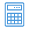, you will get the calculated results 3 in the next line with calculated result identifier >>>.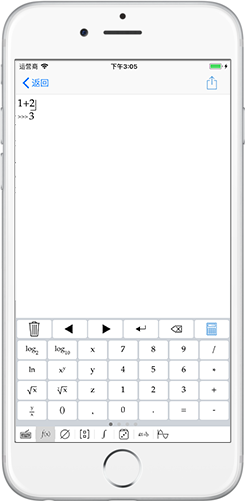2. Photo calculator
3. Step-by-step guidance
• Four arithmetic operation

$\begin{array}{l}\mathrm{1+2*\left(5*6-2\right)+8*9}\\ \mathrm{>>>1+2*\left(30-2\right)+8*9}\\ \mathrm{>>>1+2*\left(28\right)+8*9}\\ \mathrm{>>>1+56+72}\\ \mathrm{>>>57+72}\\ \mathrm{>>>129}\end{array}$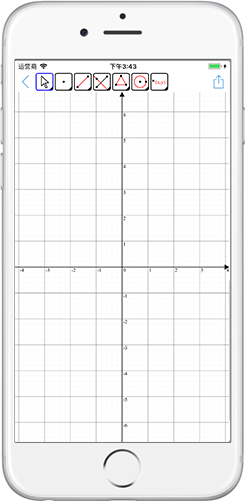•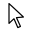Select or move the geometry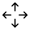Drag coordinate system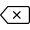Delete the selected geometry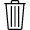Clear

•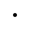Point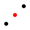Select two points to create midpoint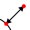Separation of attachment point from parent object

•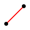Create segment with two points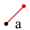Fixed length line:select a point and enter the length of the segment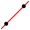Create line with two points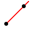Create ray with two points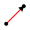Create vector with two points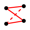Polyline:select all the vertices in turn, then click the first point

•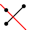Perpendicular line:select a point and a straight line to be vertical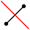Perpendicular bisector line:select two points or a line segment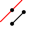Parallel line:select a point and a line to parallel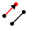Equal vector:select a point and a vector to parallel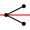Angle bisector:select three points or two straight lines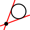Tangent line:select a point and a circle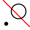Polar line:select a point and a circle

•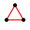Polygon:select all the vertices in turn, then click the first point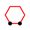Regular polygon:select two points and enter vertex number

•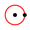Circle:select center point of the circle and a point in circlein turn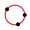Circle:select not three points on the same line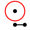Compasses:select a point to determine the center of the circle and a segment to determine the radius of the circle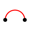Semicircle arc:select two points,draw from the starting point to the end of the counter clockwise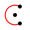Circular arc:select the center,the starting point,the end point in turn,draw from the starting point to the end of the counter clockwise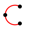Circular arc:select not three points on the same line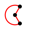Circular sector:select the center,the starting point,the end point in turnCircular sector:select not three points on the same line

• Measure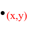Coordinate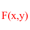Equation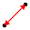Length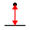Distance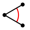Angle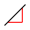Slope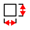Perimeter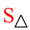Area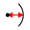Radius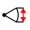Arc Length